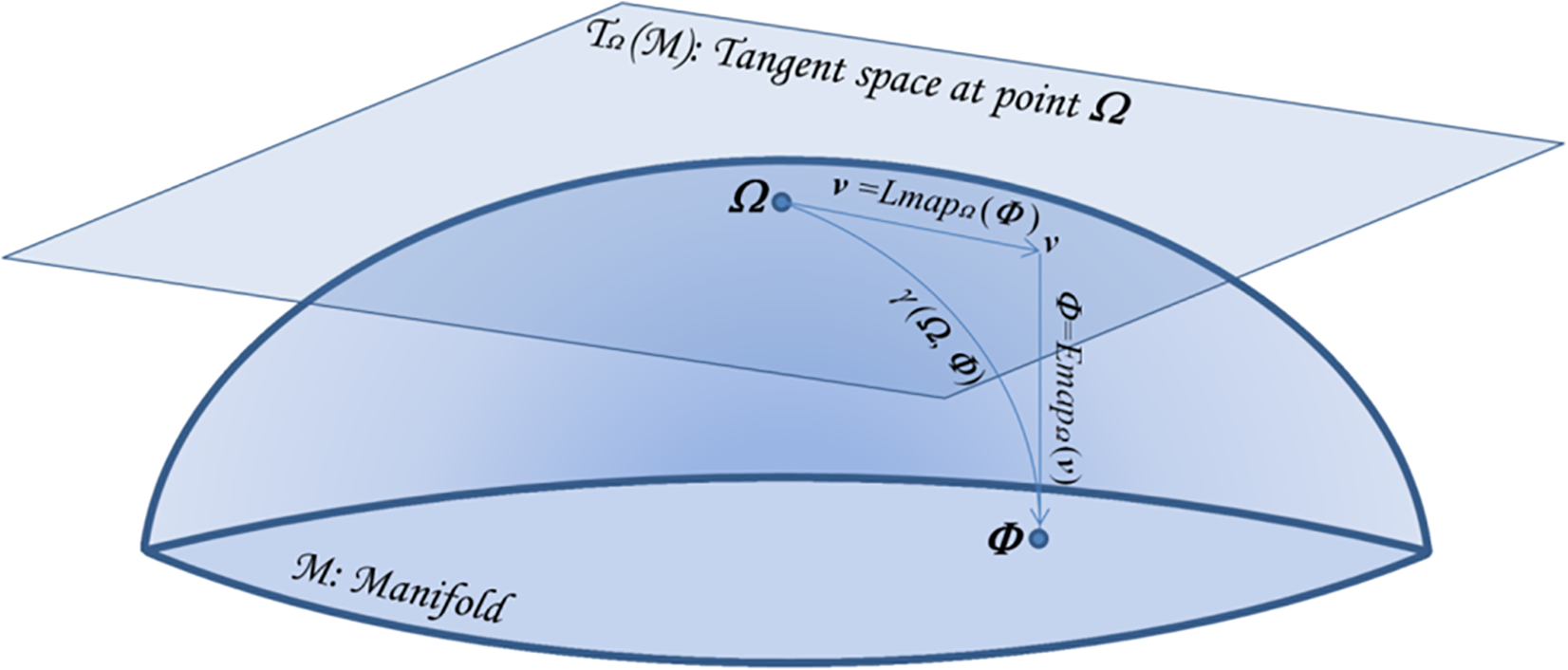We explore the connection between two problems that have arisen independently in the signal processing and related fields: the estimation of the geometric mean of a set of symmetric positive definite (SPD) matrices and their approximate joint diagonalization (AJD). Today there is a considerable interest in estimating the geometric mean of a SPD matrix set in the manifold of SPD matrices endowed with the Fisher information metric. The resulting mean has several important invariance properties and has proven very useful in diverse engineering applications such as biomedical and image data processing. While for two SPD matrices the mean has an algebraic closed form solution, for a set of more than two SPD matrices it can only be estimated by iterative algorithms. However, none of the existing iterative algorithms feature at the same time fast convergence, low computational complexity per iteration and guarantee of convergence. For this reason, recently other definitions of geometric mean based on symmetric divergence measures, such as the Bhattacharyya divergence, have been considered. The resulting means, although possibly useful in practice, do not satisfy all desirable invariance properties. In this paper we consider geometric means of covariance matrices estimated on high-dimensional time-series, assuming that the data is generated according to an instantaneous mixing model, which is very common in signal processing. We show that in these circumstances we can approximate the Fisher information geometric mean by employing an efficient AJD algorithm. Our approximation is in general much closer to the Fisher information geometric mean as compared to its competitors and verifies many invariance properties. Furthermore, convergence is guaranteed, the computational complexity is low and the convergence rate is quadratic. The accuracy of this new geometric mean approximation is demonstrated by means of simulations.